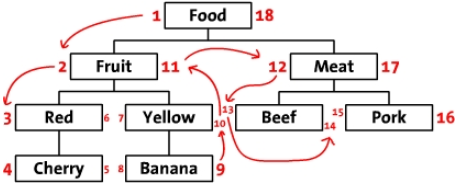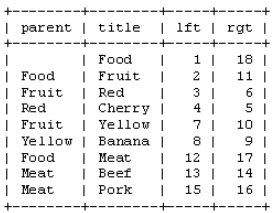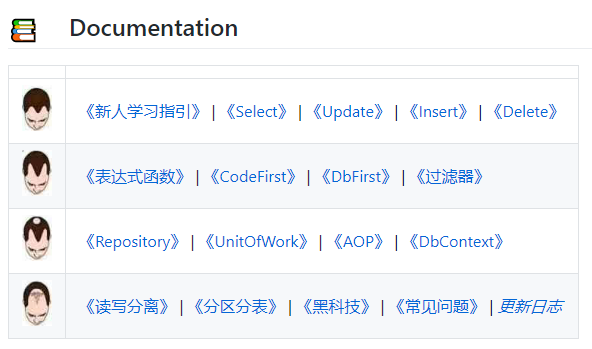# FreeSql 使用 ToTreeList/AsTreeCte 查询无限级分类表

## 关于无限级分类

无限级分类----改进前序遍历树## 引出痛点

• 第一种方案：表设计中只有 parent_id 字段，写入数据方便，困扰：查询麻烦，许多使用了 ORM 的项目被迫使用 SQL 解决该场景；
• 第二种方案：表设计中冗余子级id便于查询，困扰：添加/更新/删除的时候需要重新计算；
• 第三种方案：表设计中存储左右值编码，困扰：同上；

## 关于 FreeSql

FreeSql 是功能强大的对象关系映射技术(O/RM)，支持 .NETCore 2.1+ 或 .NETFramework 4.0+ 或 Xamarin，以 MIT 开源协议托管于 github，单元测试数量 4528个，nuget 下载量 151K，支持 MySql/SqlServer/PostgreSQL/Oracle/Sqlite/达梦/人大金仓/神州通用/Access；## 第一步：定义导航属性

FreeSql 导航属性之中，有针对父子关系的设置方式，ToTreeList/AsTreeCte 依赖该设置，如下：

public class Area
{
[Column(IsPrimary = true)]
public string Code { get; set; }

public string Name { get; set; }
public string ParentCode { get; set; }

[Navigate(nameof(ParentCode)), JsonIgnore] //JsonIgnore 是 json.net 的特性
public Area Parent { get; set; }
[Navigate(nameof(ParentCode))]
public List<Area> Childs { get; set; }
}


### 关于导航属性

fsql.Select<Area>()
.Where(a => a.Parent.Parent.Parent.Name == "中国")
.First();


fsql.Select<Area>()
.Where(a => a.Childs.AsSelect().Any(c => c.Name == "北京"))
.First();


### 添加测试数据

fsql.Delete<Area>().Where("1=1").ExecuteAffrows();
var repo = fsql.GetRepository<Area>();
repo.DbContextOptions.NoneParameter = true;
repo.Insert(new Area
{
Code = "100000",
Name = "中国",
Childs = new List<Area>(new[] {
new Area
{
Code = "110000",
Name = "北京",
Childs = new List<Area>(new[] {
new Area{ Code="110100", Name = "北京市" },
new Area{ Code="110101", Name = "东城区" },
})
}
})
});


## 第二步：使用 ToTreeList 返回树型数据

var t1 = fsql.Select<Area>().ToTreeList();
Assert.Single(t1);
Assert.Equal("100000", t1.Code);
Assert.Single(t1.Childs);
Assert.Equal("110000", t1.Childs.Code);
Assert.Equal(2, t1.Childs.Childs.Count);
Assert.Equal("110100", t1.Childs.Childs.Code);
Assert.Equal("110101", t1.Childs.Childs.Code);


[
{
"ParentCode": null,
"Childs": [
{
"ParentCode": "100000",
"Childs": [
{
"ParentCode": "110000",
"Childs": [],
"Code": "110100",
"Name": "北京市"
},
{
"ParentCode": "110000",
"Childs": [],
"Code": "110101",
"Name": "东城区"
}
],
"Code": "110000",
"Name": "北京"
}
],
"Code": "100000",
"Name": "中国"
}
]


## 第三步：使用 AsTreeCte 递归查询

(可选) pathSelector 路径内容选择，可以设置查询返回：中国 -> 北京 -> 东城区
(可选) up false(默认)：由父级向子级的递归查询，true：由子级向父级的递归查询
(可选) pathSeparator 设置 pathSelector 的连接符，默认：->
(可选) level 设置递归层级

var t2 = fsql.Select<Area>()
.Where(a => a.Name == "中国")
.AsTreeCte() //查询 中国 下的所有记录
.OrderBy(a => a.Code)
.ToTreeList(); //非必须，也可以使用 ToList（见姿势二）
Assert.Single(t2);
Assert.Equal("100000", t2.Code);
Assert.Single(t2.Childs);
Assert.Equal("110000", t2.Childs.Code);
Assert.Equal(2, t2.Childs.Childs.Count);
Assert.Equal("110100", t2.Childs.Childs.Code);
Assert.Equal("110101", t2.Childs.Childs.Code);
// WITH "as_tree_cte"
// as
// (
// SELECT 0 as cte_level, a."Code", a."Name", a."ParentCode"
// FROM "Area" a
// WHERE (a."Name" = '中国')

// union all

// SELECT wct1.cte_level + 1 as cte_level, wct2."Code", wct2."Name", wct2."ParentCode"
// FROM "as_tree_cte" wct1
// INNER JOIN "Area" wct2 ON wct2."ParentCode" = wct1."Code"
// )
// SELECT a."Code", a."Name", a."ParentCode"
// FROM "as_tree_cte" a
// ORDER BY a."Code"


var t3 = fsql.Select<Area>()
.Where(a => a.Name == "中国")
.AsTreeCte()
.OrderBy(a => a.Code)
.ToList();
Assert.Equal(4, t3.Count);
Assert.Equal("100000", t3.Code);
Assert.Equal("110000", t3.Code);
Assert.Equal("110100", t3.Code);
Assert.Equal("110101", t3.Code);
//执行的 SQL 与姿势一相同


var t4 = fsql.Select<Area>()
.Where(a => a.Name == "中国")
.AsTreeCte(a => a.Name + "[" + a.Code + "]")
.OrderBy(a => a.Code)
.ToList(a => new {
item = a,
level = Convert.ToInt32("a.cte_level"),
path = "a.cte_path"
});
Assert.Equal(4, t4.Count);
Assert.Equal("100000", t4.item.Code);
Assert.Equal("110000", t4.item.Code);
Assert.Equal("110100", t4.item.Code);
Assert.Equal("110101", t4.item.Code);
Assert.Equal("中国", t4.path);
Assert.Equal("中国 -> 北京", t4.path);
Assert.Equal("中国 -> 北京 -> 北京市", t4.path);
Assert.Equal("中国 -> 北京 -> 东城区", t4.path);
// WITH "as_tree_cte"
// as
// (
// SELECT 0 as cte_level, a."Name" || '[' || a."Code" || ']' as cte_path, a."Code", a."Name", a."ParentCode"
// FROM "Area" a
// WHERE (a."Name" = '中国')

// union all

// SELECT wct1.cte_level + 1 as cte_level, wct1.cte_path || ' -> ' || wct2."Name" || '[' || wct2."Code" || ']' as cte_path, wct2."Code", wct2."Name", wct2."ParentCode"
// FROM "as_tree_cte" wct1
// INNER JOIN "Area" wct2 ON wct2."ParentCode" = wct1."Code"
// )
// SELECT a."Code" as1, a."Name" as2, a."ParentCode" as5, a.cte_level as6, a.cte_path as7
// FROM "as_tree_cte" a
// ORDER BY a."Code"


posted @ 2020-06-28 06:41  FreeSql  阅读(2498)  评论(19编辑  收藏  举报